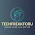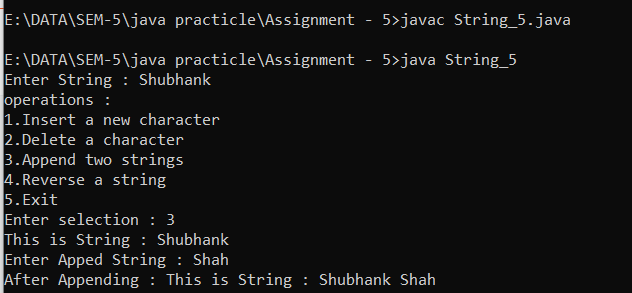# Character Up

• The orientation for a displayed character string is set according to the direction of the character up vector.

• char_up (x, y)

• Parameter in the function is assigned two values for x and y value components.

• Text is then displayed so that the orientation of character from baseline to topline is in the direction of the "up vector".

• E.x - char_up (1, 1) the direction is in the up vector is 45 degree and text would be displayed as given below:

• A producer for orienting text rotates character so that the side of character bodies, from baseline to cap line is aligned with the up vector.

# Routine Instructions

setcharup (dx, dy)

{

s = sqrt (pow (dx, 2) + pow (dy, 2))

if (s < roundoff) then

Error: "Very Small Value"

ds = (abs (cw * dy) + abs (ch * dx)) / s

ts = ds * (1 + charsep)

xcharsp = ts * (dy / s)

ycharsp = ts * (-dx / s)

}

text (String)

{

len = strlen (String);

x = df - penx;

y = df - peny;

for (i = 1 to len)

chr = String [i]

op = chr

op = -op

dis_file_enter (op);

df_penx += xcharsp

df_peny += ycharsp

move_abs (x, y)

}

interpret (start, count)

{

for (n = start to count)

getpoint (n, op, x, y);

if (op < -31 && op > -127)

dochar (op, x, y);

else if (op == 1)

domove (x, y);

else if (op == 2)

doline (x, y);

else

"op code error"

}

dochar (op, x, y)

{

frame_penx = max (ws, min (we, x * w + ws))

frame_peny = max (hs, min (he, y * h + hs))

op = -op

chr = op

switch (chr)

case 'h' : genh (frame_penx, frame_peny);

case 'e' : genh (frame_penx, frame_peny);

case 'l' : genh (frame_penx, frame_peny);

case 'l' : genh (frame_penx, frame_peny);

case 'o' : genh (frame_penx, frame_peny);

}

initialize ()

{

old initialize ()

ch = 0.1;

cw = 0.1;

char_sep = 0;

set_charup (1, 0) / (0, 1);

}

main ()

{

char_string  = {'h','e','l','l','o'};

initialize ();

new_frame ();

text (string);

make_picture_current ();

}

Illustrate the Bresenham Algorithm For Endpoints (-1, 7) to (5, 9)

## Thank you for visiting my blog :)

1.In this code which routine is used to place instructions for printing a string into display file?

1.In this code, "text" routine is used to place instructions for printing a string into display file.

here, how it works:

"text" routine will get opcodes of char and store it into display file and "make_pic_current()" will use it and "dochar()" will call opcodes or char function and in last it will print your string...